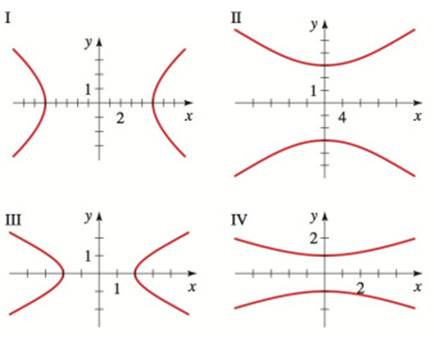Chapter 12.3, Problem 7E### Algebra and Trigonometry (MindTap ...

4th Edition
James Stewart + 2 others
ISBN: 9781305071742

#### Solutions

Chapter
Section### Algebra and Trigonometry (MindTap ...

4th Edition
James Stewart + 2 others
ISBN: 9781305071742
Textbook Problem

# SKILLSGraphs of Hyperbolas Match the equation with the graphs labeled I − I V . Give reasons for your answers. 16 y 2 − x 2 = 144To determine

The graph for the given hyperbola 16y2x2=144.

Explanation

Given:

Equation of hyperbola,

16y2x2=144

Approach:

The form of hyperbola equation with vertical transverse is given by,

y2a2x2b2=1 or y2a2+x2b2=1

Calculation:

The given equation can be converted into hyperbola equation as follows:

16y2x2=1441144(16y2x2)</

### Still sussing out bartleby?

Check out a sample textbook solution.

See a sample solution

#### The Solution to Your Study Problems

Bartleby provides explanations to thousands of textbook problems written by our experts, many with advanced degrees!

Get Started